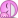# Motion Eqaution and Newtons Law Problem?

A projectile has been launched and moves according to the equations: y = 6.00 + 3.45t − 7.20t2 m, and x = 17.0t m. (a) Do these equations describe an object launched on Earth? (b) Assume the object is a slide rule of mass 0.207 kg. What force is being exerted on it?

I know part a is no, since the acceleration isnt 9.8, but I have no idea how to do part b? any help?

F=ma

Do you understand what each of the terms in your equation of motion mean? Remember that $$s=s_{i}+v_{i}t+\frac{1}{2}at^{2}$$DDDDDD

Last edited:
rock.freak667
Homework Helper
I know part a is no, since the acceleration isnt 9.8, but I have no idea how to do part b? any help?

So if y is displacement, how does one find velocity and hence acceleration?

Now use the message below.

F=ma

ok, I see just take the 2nd derivative of y and x and multiply by mass

thanks guys